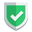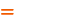我们相信：世界是美好的，你是我也是。平行空间的世界里面，不同版本的生活也在继续...

## 基本数据

• 它的实际情况是：`[0 0 0 1 1 1]`
• 大数据模型根据机器学习的结果预测的结果是：`[1 1 1 0 0 1]`

## 基础分类

tp正确的阳性结论11准确有问题，还被预测出来了
fp虚假的阳性结论10不准确没问题，却被错误的预测出来了
tn正确的阴性结论00准确没问题，同时也确实被预测出来
fn虚假的阴性结论01不准确有问题，但是没被预测出来

``````tp = sum( (y_pred == 1) & (y_true == 1) )
fp = sum( (y_pred == 1) & (y_true == 0) )
tn = sum( (y_pred == 0) & (y_true == 0) )
fn = sum( (y_pred == 0) & (y_true == 1) )``````

## 查准率 precision_score

• 阳性有关的样本中，预测准确的样本比率。【查准率】
• 阴性有关的样本中，预测准确的样本比率。
• 所有样本中，预测准确的样本比率。

``````p = tp / (tp + fp)
p_n = tn / (tn + fn)
p_all = (tp + tn) / (tp + fp + tn + fn)``````

``````官方准确率: 0.25

``````p = precision_score(y_true, y_pred)
p_ = (tp / (tp + fp)) if (tp + fp > 0) else 0``````

## 召回率`recall_score`

• 预测为阳性，[==>]真实阳性，准确(tp)。真实阴性，不准确(fp)。
• 预测为阴性，真实阴性，准确(tn)。[==>]真实阳性，不准确(fn)。

``r = tp / (tp + fn)``

``````r = recall_score(y_true, y_pred)
r_ = (tp / (tp + fn)) if (tp + fn > 0) else 0``````

## f1得分`f1_score`

`f1`得分是综合上述两者得分的，`f1`得分越低，证明模型选的越不好。计算方法是：

``f1 = 2 * p * r / (p + r)``

``````f1 = f1_score(y_true, y_pred)
f1_ = 2 * p_ * r_ / (p_ + r_) if (p_ + r_ > 0) else 0``````

## 测试代码

``````from sklearn.metrics import precision_score, recall_score, f1_score
import numpy as np

def analyze(y_true, y_pred):
print("实际值", y_true)
print("预测值", y_pred)
print("对不对", y_true == y_pred)

tp = sum((y_pred == 1) & (y_true == 1))
fp = sum((y_pred == 1) & (y_true == 0))
tn = sum((y_pred == 0) & (y_true == 0))
fn = sum((y_pred == 0) & (y_true == 1))

print("有问题被发现了（tp）:%s" % tp)
print("有问题没被发现（fn）:%s" % fn)
print("没有问题被误诊（fp）:%s" % fp)
print("放心啥事都没有（tn）:%s" % tn)

p = precision_score(y_true, y_pred)
r = recall_score(y_true, y_pred)
f1 = f1_score(y_true, y_pred)

print("官方准确率:%s" % p)
print("召回率:%s" % r)
print("F1得分:%s" % f1)

p_ = (tp / (tp + fp)) if (tp + fp > 0) else 0
r_ = (tp / (tp + fn)) if (tp + fn > 0) else 0
f1_ = 2 * p_ * r_ / (p_ + r_) if (p_ + r_ > 0) else 0

print("准确率自定义:%s" % p_)
print("召回率自定义:%s" % r_)
print("F1得分自定义:%s" % f1_)

p_2 = (tn / (tn + fn)) if (tn + fn > 0) else 0
p_3 = ((tp + tn) / (tp + fp + tn + fn)) if (tp + fp + tn + fn > 0) else 0
print("阳性准确率[tp / tp + fp]:%s" % p_)
print("阴性准确率[tn / tn + fp]:%s" % p_2)
print("整体准确率[(tp + tn) / (tp + fp + tn + fn)]:%s" % p_3)

def analyze2(y_test, predictions):
y_true = np.array(y_test)       # 1 有问题的，应该被召回  0 没有问题的，不应该被召回
y_pred = np.array(predictions)  # 1 预测为有问题，被召回  0 预测为没有问题，没有被召回
analyze(y_true, y_pred)
print("=" * 20)``````
``analyze2([0, 0, 0, 1, 1, 1], [1, 1, 1, 0, 0, 1])``

``````实际值 [0 0 0 1 1 1]

F1得分:0.28571428571428575

F1得分自定义:0.28571428571428575

## 结束语如果本文对您有帮助，或者节约了您的时间，欢迎打赏瓶饮料，建立下友谊关系。本博客不欢迎：各种镜像采集行为。请尊重原创文章内容，转载请保留作者链接。【福利】 腾讯云最新爆款活动！1核2G云服务器首年50元！【源码】本文代码片段及相关软件，请点此获取更多信息【绝密】秘籍文章入口，仅传授于有缘之人python    sklearn